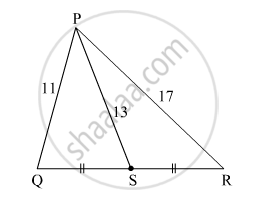Share

# In ∆Pqr, Point S is the Midpoint of Side Qr. If Pq = 11, Pr = 17, Ps =13, Find Qr. - Geometry

ConceptPythagoras Theorem

#### Question

In ∆PQR, point S is the midpoint of side QR. If PQ = 11, PR = 17, PS =13, find QR.

#### SolutionIn ∆PQR, point S is the midpoint of side QR.

$QS = SR = \frac{1}{2}QR$

${PQ}^2 + {PR}^2 = 2 {PS}^2 + 2 {QS}^2 \left( \text{by Apollonius theorem} \right)$

$\Rightarrow {11}^2 + {17}^2 = 2 \left( 13 \right)^2 + 2 {QS}^2$

$\Rightarrow 121 + 289 = 2\left( 169 \right) + 2 {QS}^2$

$\Rightarrow 410 = 338 + 2 {QS}^2$

$\Rightarrow 2 {QS}^2 = 410 - 338$

$\Rightarrow 2 {QS}^2 = 72$

$\Rightarrow {QS}^2 = 36$

$\Rightarrow QS = 6$

$\therefore QR = 2 \times QS$

$= 2 \times 6$

$= 12$

Hence, QR = 12.

Is there an error in this question or solution?

#### Video TutorialsVIEW ALL 

Solution In ∆Pqr, Point S is the Midpoint of Side Qr. If Pq = 11, Pr = 17, Ps =13, Find Qr. Concept: Pythagoras Theorem.
S